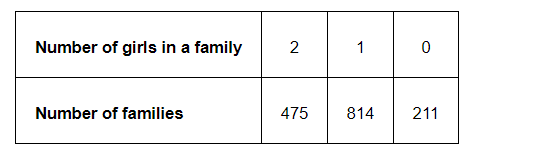# 1500 families with 2 children were selected randomly,

Question.

1500 families with 2 children were selected randomly, and the following data were recorded:Compute the probability of a family, chosen at random, having

(i) 2 girls

(ii) 1 girl

(iii) No girl

Also check whether the sum of these probabilities is 1.

Solution:

Total number of families = 475 + 814 + 211

= 1500

(i) Number of families having 2 girls $=475$

$P_{1}($ a randomly chosen family has 2 girls $)=\frac{\text { Number of families having } 2 \text { girls }}{\text { Total number of families }}$

=\frac{475}{1500}=\frac{19}{60}

(ii) Number of families having 1 girl = 814

$P_{2}$ (a randomly chosen family has 1 girl) $=\frac{\text { Number of families having } 1 \text { girl }}{\text { Total number of families }}$

$=\frac{814}{1500}=\frac{407}{750}$

(iii) Number of families having no girl = 211

$P_{3}($ a randomly chosen family has no girl $)=\frac{\text { Number of families having no girl }}{\text { Total number of families }}$

$=\frac{211}{1500}$

Sum of all these probabilities $=\frac{19}{60}+\frac{407}{750}+\frac{211}{1500}$

$=\frac{475+814+211}{1500}$

$=\frac{1500}{1500}=1$

Therefore, the sum of all these probabilities is 1.